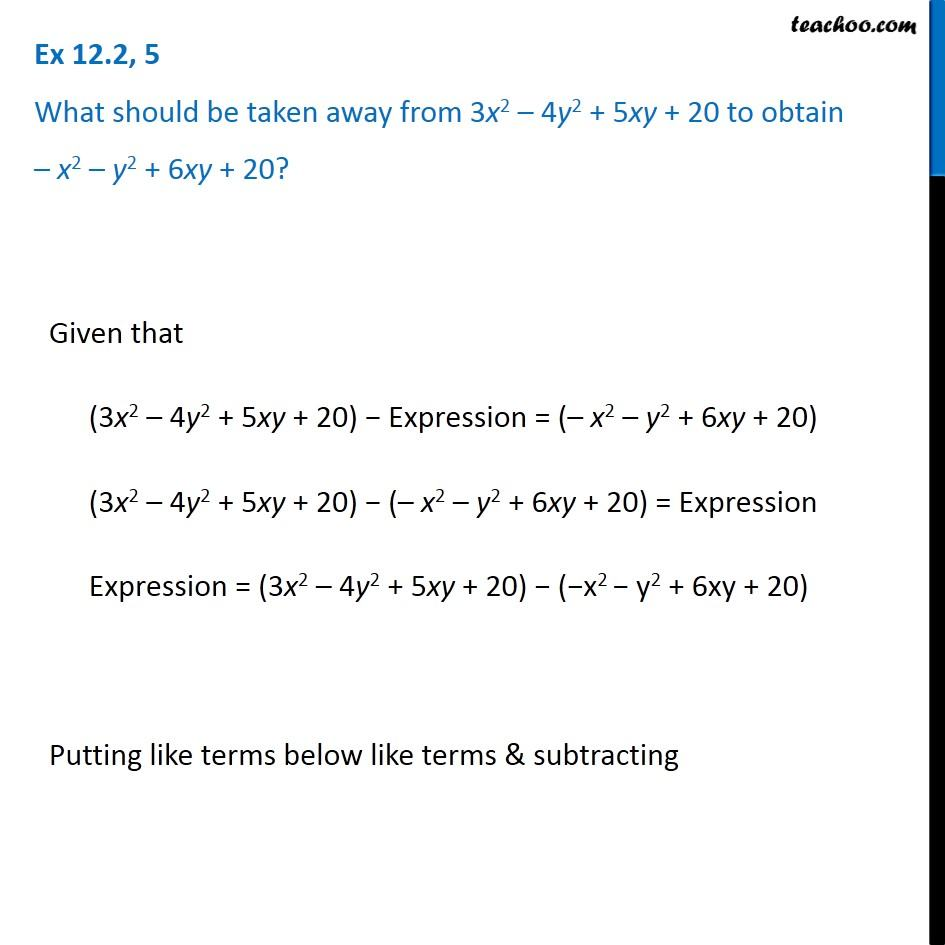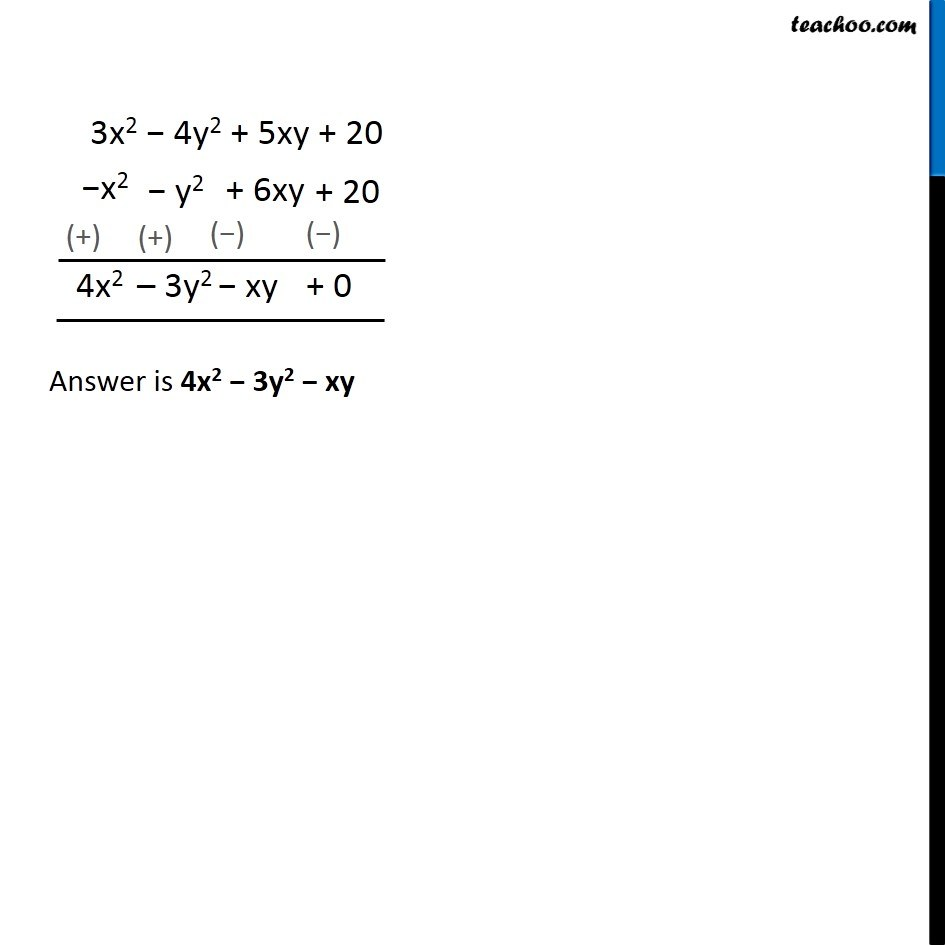Adding and Subtracting Like terms - Worksheet

Chapter 10 Class 7 Algebraic Expressions
Serial order wiseLearn in your speed, with individual attention - Teachoo Maths 1-on-1 Class

### Transcript

Question 5 What should be taken away from 3x2 – 4y2 + 5xy + 20 to obtain – x2 – y2 + 6xy + 20? Given that (3x2 – 4y2 + 5xy + 20) − Expression = (– x2 – y2 + 6xy + 20) (3x2 – 4y2 + 5xy + 20) − (– x2 – y2 + 6xy + 20) = Expression Expression = (3x2 – 4y2 + 5xy + 20) − (−x2 − y2 + 6xy + 20) Putting like terms below like terms & subtracting Answer is 4x2 − 3y2 − xy